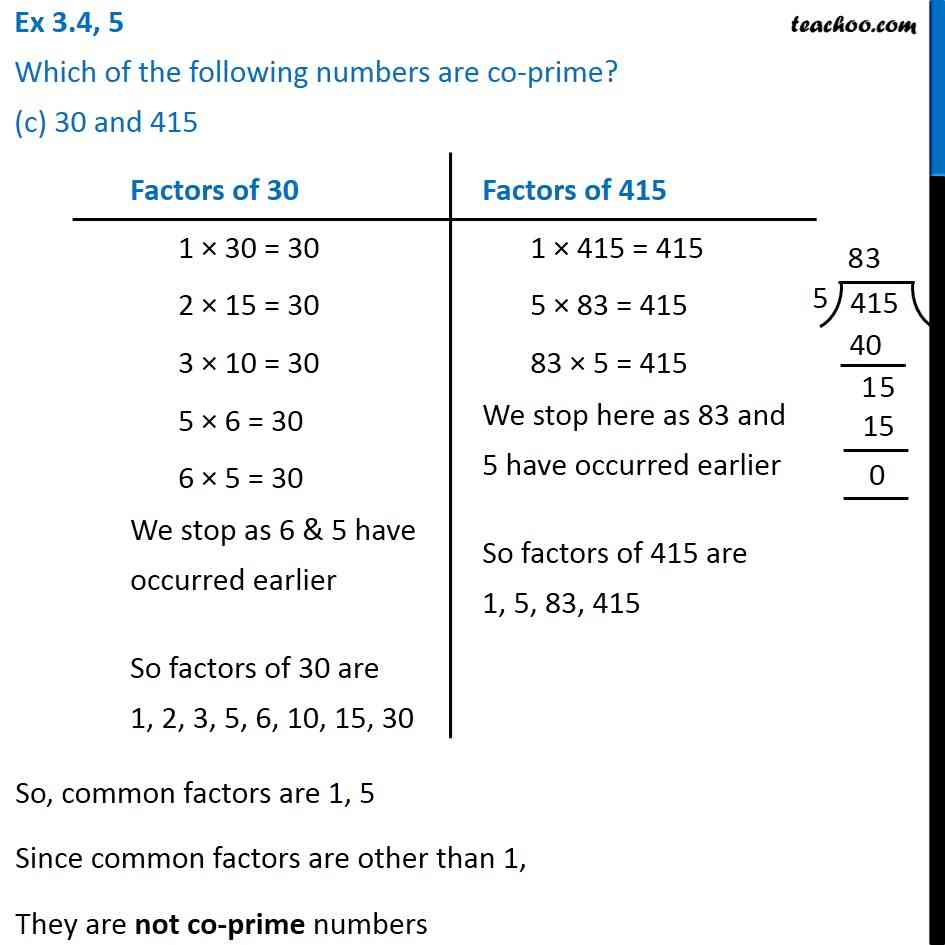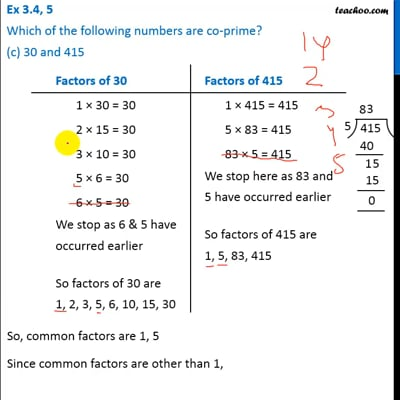Ex 3.4

Chapter 3 Class 6 Playing with Numbers
Serial order wiseThis video is only available for Teachoo black users

Learn in your speed, with individual attention - Teachoo Maths 1-on-1 Class

### Transcript

Ex 3.4, 5 Which of the following numbers are co-prime? (c) 30 and 415 Factors of 30 1 × 30 = 30 2 × 15 = 30 3 × 10 = 30 5 × 6 = 30 6 × 5 = 30 We stop as 6 & 5 have occurred earlier So factors of 30 are 1, 2, 3, 5, 6, 10, 15, 30 Factors of 415 1 × 415 = 415 5 × 83 = 415 83 × 5 = 415 We stop here as 83 and 5 have occurred earlier So factors of 415 are 1, 5, 83, 415 So, common factors are 1, 5 Since common factors are other than 1, They are not co-prime numbers